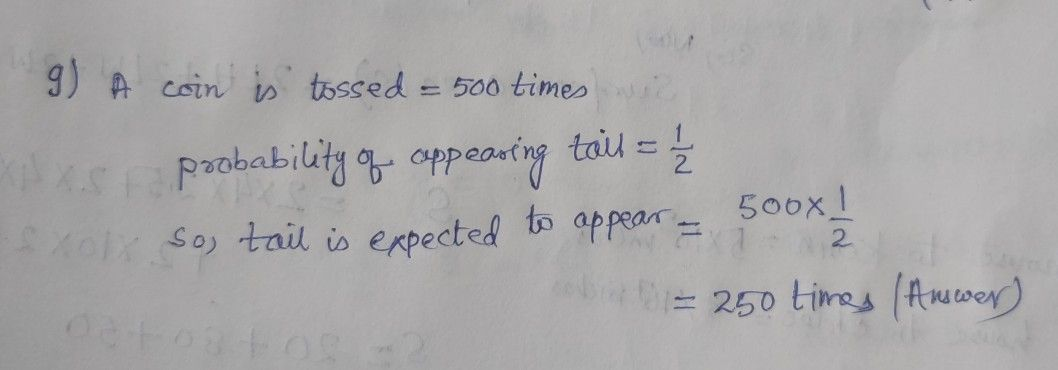Symbol
Problem$9.9\right)$ If a coin is tossed $500$ times, the tail is expected to appear Full Marks $:1$ $500$ times $250$ times $\square$ $0$ 0 times $\square$ $100$ times
7th-9th grade
Probability and Statistics
SolutionQanda teacher - Mashroorkindly evaluate
please give some extra Coins as thank you gift
if any doubts kindly ask will clarifyStudent
Sir, I hv a doubt, can it be 500, since it's not guaranteed both the events to be occurring equal number of times.Qanda teacher - Mashroor
yes, it's should be 500 also
if any doubts kindly ask will clarifyStudent
Sir then wat will be the exact answr, is it 500?
??Qanda teacher - Mashroor
here, 250 times is the exact answer
if any further doubts kindly ask will clarifyStudent
Ok sir